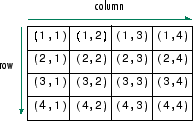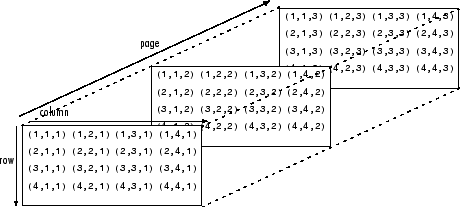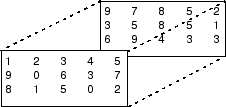Main Content

# Multidimensional Arrays

A multidimensional array in MATLAB® is an array with more than two dimensions. In a matrix, the two dimensions are represented by rows and columns.Each element is defined by two subscripts, the row index and the column index. Multidimensional arrays are an extension of 2-D matrices and use additional subscripts for indexing. A 3-D array, for example, uses three subscripts. The first two are just like a matrix, but the third dimension represents pages or sheets of elements.### Creating Multidimensional Arrays

You can create a multidimensional array by creating a 2-D matrix first, and then extending it. For example, first define a 3-by-3 matrix as the first page in a 3-D array.

`A = [1 2 3; 4 5 6; 7 8 9]`
```A = 3×3 1 2 3 4 5 6 7 8 9 ```

Now add a second page. To do this, assign another 3-by-3 matrix to the index value 2 in the third dimension. The syntax `A(:,:,2)` uses a colon in the first and second dimensions to include all rows and all columns from the right-hand side of the assignment.

`A(:,:,2) = [10 11 12; 13 14 15; 16 17 18]`
```A = A(:,:,1) = 1 2 3 4 5 6 7 8 9 A(:,:,2) = 10 11 12 13 14 15 16 17 18 ```

The `cat` function can be a useful tool for building multidimensional arrays. For example, create a new 3-D array `B` by concatenating `A` with a third page. The first argument indicates which dimension to concatenate along.

`B = cat(3,A,[3 2 1; 0 9 8; 5 3 7])`
```B = B(:,:,1) = 1 2 3 4 5 6 7 8 9 B(:,:,2) = 10 11 12 13 14 15 16 17 18 B(:,:,3) = 3 2 1 0 9 8 5 3 7 ```

Another way to quickly expand a multidimensional array is by assigning a single element to an entire page. For example, add a fourth page to `B` that contains all zeros.

`B(:,:,4) = 0`
```B = B(:,:,1) = 1 2 3 4 5 6 7 8 9 B(:,:,2) = 10 11 12 13 14 15 16 17 18 B(:,:,3) = 3 2 1 0 9 8 5 3 7 B(:,:,4) = 0 0 0 0 0 0 0 0 0 ```

### Accessing Elements

To access elements in a multidimensional array, use integer subscripts just as you would for vectors and matrices. For example, find the 1,2,2 element of `A`, which is in the first row, second column, and second page of `A`.

`A`
```A = A(:,:,1) = 1 2 3 4 5 6 7 8 9 A(:,:,2) = 10 11 12 13 14 15 16 17 18 ```
`elA = A(1,2,2)`
```elA = 11 ```

Use the index vector `[1 3]` in the second dimension to access only the first and last columns of each page of `A`.

`C = A(:,[1 3],:)`
```C = C(:,:,1) = 1 3 4 6 7 9 C(:,:,2) = 10 12 13 15 16 18 ```

To find the second and third rows of each page, use the colon operator to create your index vector.

`D = A(2:3,:,:)`
```D = D(:,:,1) = 4 5 6 7 8 9 D(:,:,2) = 13 14 15 16 17 18 ```

### Manipulating Arrays

Elements of multidimensional arrays can be moved around in many ways, similar to vectors and matrices. `reshape`, `permute`, and `squeeze` are useful functions for rearranging elements. Consider a 3-D array with two pages.Reshaping a multidimensional array can be useful for performing certain operations or visualizing the data. Use the `reshape` function to rearrange the elements of the 3-D array into a 6-by-5 matrix.

```A = [1 2 3 4 5; 9 0 6 3 7; 8 1 5 0 2]; A(:,:,2) = [9 7 8 5 2; 3 5 8 5 1; 6 9 4 3 3]; B = reshape(A,[6 5])```
```B = 6×5 1 3 5 7 5 9 6 7 5 5 8 5 2 9 3 2 4 9 8 2 0 3 3 8 1 1 0 6 4 3 ```

`reshape` operates columnwise, creating the new matrix by taking consecutive elements down each column of `A`, starting with the first page then moving to the second page.

Permutations are used to rearrange the order of the dimensions of an array. Consider a 3-D array `M`.

```M(:,:,1) = [1 2 3; 4 5 6; 7 8 9]; M(:,:,2) = [0 5 4; 2 7 6; 9 3 1]```
```M = M(:,:,1) = 1 2 3 4 5 6 7 8 9 M(:,:,2) = 0 5 4 2 7 6 9 3 1 ```

Use the `permute` function to interchange row and column subscripts on each page by specifying the order of dimensions in the second argument. The original rows of `M` are now columns, and the columns are now rows.

`P1 = permute(M,[2 1 3])`
```P1 = P1(:,:,1) = 1 4 7 2 5 8 3 6 9 P1(:,:,2) = 0 2 9 5 7 3 4 6 1 ```

Similarly, interchange row and page subscripts of `M`.

`P2 = permute(M,[3 2 1])`
```P2 = P2(:,:,1) = 1 2 3 0 5 4 P2(:,:,2) = 4 5 6 2 7 6 P2(:,:,3) = 7 8 9 9 3 1 ```

When working with multidimensional arrays, you might encounter one that has an unnecessary dimension of length 1. The `squeeze` function performs another type of manipulation that eliminates dimensions of length 1. For example, use the `repmat` function to create a 2-by-3-by-1-by-4 array whose elements are each 5, and whose third dimension has length 1.

`A = repmat(5,[2 3 1 4])`
```A = A(:,:,1,1) = 5 5 5 5 5 5 A(:,:,1,2) = 5 5 5 5 5 5 A(:,:,1,3) = 5 5 5 5 5 5 A(:,:,1,4) = 5 5 5 5 5 5 ```
`szA = size(A)`
```szA = 1×4 2 3 1 4 ```
`numdimsA = ndims(A)`
```numdimsA = 4 ```

Use the `squeeze` function to remove the third dimension, resulting in a 3-D array.

`B = squeeze(A)`
```B = B(:,:,1) = 5 5 5 5 5 5 B(:,:,2) = 5 5 5 5 5 5 B(:,:,3) = 5 5 5 5 5 5 B(:,:,4) = 5 5 5 5 5 5 ```
`szB = size(B)`
```szB = 1×3 2 3 4 ```
`numdimsB = ndims(B)`
```numdimsB = 3 ```

Download ebook